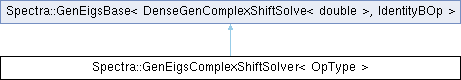Spectra  1.0.1 Header-only C++ Library for Large Scale Eigenvalue Problems
Spectra::GenEigsComplexShiftSolver< OpType > Class Template Reference

#include <Spectra/GenEigsComplexShiftSolver.h>

Inheritance diagram for Spectra::GenEigsComplexShiftSolver< OpType >:## Public Member Functions

GenEigsComplexShiftSolver (OpType &op, Index nev, Index ncv, const Scalar &sigmar, const Scalar &sigmai)Public Member Functions inherited from Spectra::GenEigsBase< DenseGenComplexShiftSolve< double >, IdentityBOp >
void init (const Scalar *init_resid)

void init ()

Index compute (SortRule selection=SortRule::LargestMagn, Index maxit=1000, Scalar tol=1e-10, SortRule sorting=SortRule::LargestMagn)

CompInfo info () const

Index num_iterations () const

Index num_operations () const

ComplexVector eigenvalues () const

ComplexMatrix eigenvectors (Index nvec) const

ComplexMatrix eigenvectors () const

## Detailed Description

### template<typename OpType = DenseGenComplexShiftSolve<double>> class Spectra::GenEigsComplexShiftSolver< OpType >

This class implements the eigen solver for general real matrices with a complex shift value in the shift-and-invert mode. The background knowledge of the shift-and-invert mode can be found in the documentation of the SymEigsShiftSolver class.

Template Parameters
 OpType The name of the matrix operation class. Users could either use the wrapper classes such as DenseGenComplexShiftSolve and SparseGenComplexShiftSolve, or define their own that implements the type definition Scalar and all the public member functions as in DenseGenComplexShiftSolve.

Definition at line 33 of file GenEigsComplexShiftSolver.h.

## ◆ GenEigsComplexShiftSolver()

template<typename OpType = DenseGenComplexShiftSolve<double>>
 Spectra::GenEigsComplexShiftSolver< OpType >::GenEigsComplexShiftSolver ( OpType & op, Index nev, Index ncv, const Scalar & sigmar, const Scalar & sigmai )
inline

Constructor to create a eigen solver object using the shift-and-invert mode.

Parameters
 op The matrix operation object that implements the complex shift-solve operation of $$A$$: calculating $$\mathrm{Re}\{(A-\sigma I)^{-1}v\}$$ for any vector $$v$$. Users could either create the object from the wrapper class such as DenseGenComplexShiftSolve, or define their own that implements all the public members as in DenseGenComplexShiftSolve. nev Number of eigenvalues requested. This should satisfy $$1\le nev \le n-2$$, where $$n$$ is the size of matrix. ncv Parameter that controls the convergence speed of the algorithm. Typically a larger ncv means faster convergence, but it may also result in greater memory use and more matrix operations in each iteration. This parameter must satisfy $$nev+2 \le ncv \le n$$, and is advised to take $$ncv \ge 2\cdot nev + 1$$. sigmar The real part of the shift. sigmai The imaginary part of the shift.

Definition at line 149 of file GenEigsComplexShiftSolver.h.

The documentation for this class was generated from the following file: